# WO2010046968A1 - Diagnostic device and diagnostic method for concrete structure - Google Patents

Diagnostic device and diagnostic method for concrete structure Download PDF

## Info

Publication number
WO2010046968A1
WO2010046968A1 PCT/JP2008/069034 JP2008069034W WO2010046968A1 WO 2010046968 A1 WO2010046968 A1 WO 2010046968A1 JP 2008069034 W JP2008069034 W JP 2008069034W WO 2010046968 A1 WO2010046968 A1 WO 2010046968A1
Authority
WO
WIPO (PCT)
Prior art keywords
surface
concrete structure
wavelength
absorbance
concentration
Prior art date
Application number
PCT/JP2008/069034
Other languages
French (fr)
Japanese (ja)
Inventor

Original Assignee

Priority date (The priority date is an assumption and is not a legal conclusion. Google has not performed a legal analysis and makes no representation as to the accuracy of the date listed.)
Filing date
Publication date
Application filed by 西日本高速道路エンジニアリング四国株式会社 filed Critical 西日本高速道路エンジニアリング四国株式会社
Priority to PCT/JP2008/069034 priority Critical patent/WO2010046968A1/en
Publication of WO2010046968A1 publication Critical patent/WO2010046968A1/en

## Images

•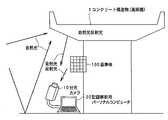•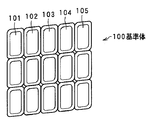•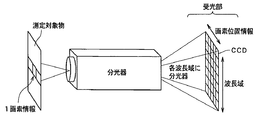•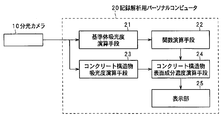•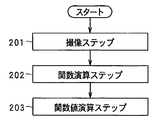•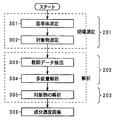•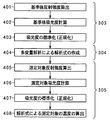•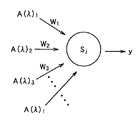•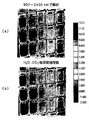•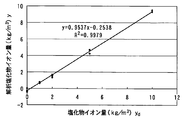•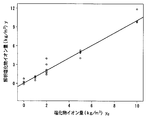•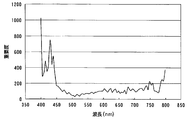•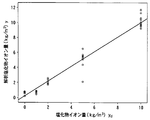•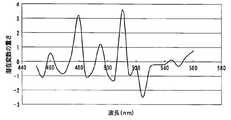•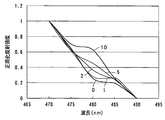•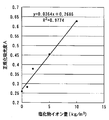•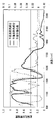•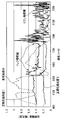•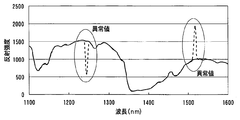## Classifications

• GPHYSICS
• G01MEASURING; TESTING
• G01NINVESTIGATING OR ANALYSING MATERIALS BY DETERMINING THEIR CHEMICAL OR PHYSICAL PROPERTIES
• G01N21/00Investigating or analysing materials by the use of optical means, i.e. using infra-red, visible or ultra-violet light
• G01N21/17Systems in which incident light is modified in accordance with the properties of the material investigated
• G01N21/25Colour; Spectral properties, i.e. comparison of effect of material on the light at two or more different wavelengths or wavelength bands
• G01N21/27Colour; Spectral properties, i.e. comparison of effect of material on the light at two or more different wavelengths or wavelength bands using photo-electric detection ; circuits for computing concentration
• G01N21/274Calibration, base line adjustment, drift correction
• GPHYSICS
• G01MEASURING; TESTING
• G01NINVESTIGATING OR ANALYSING MATERIALS BY DETERMINING THEIR CHEMICAL OR PHYSICAL PROPERTIES
• G01N21/00Investigating or analysing materials by the use of optical means, i.e. using infra-red, visible or ultra-violet light
• G01N21/17Systems in which incident light is modified in accordance with the properties of the material investigated
• G01N21/25Colour; Spectral properties, i.e. comparison of effect of material on the light at two or more different wavelengths or wavelength bands

## Abstract

This aims to provide a diagnostic device and a diagnostic method for a concrete structure, which can measure the surface constitution concentration of an outdoor concrete structure practically precisely in noncontact and nondestructive manners. In the diagnostic method, a reference body having individual components, in which the concentrations of constituents existing on the surface of the concrete structure are made different to individual levels, is arranged under the environment, in which the surface of the reference body is irradiated with the natural light having the same or substantially the same spectrum as that of a natural light toemit the surface of the concrete structure to be diagnosed. The light, which is reflected on the surface of the concrete structure and the surface of the reference body, is separated into individual wavelengths and photographed, under the environment, in which the surface of the concrete structure and the surface of the reference body are irradiated with the natural light of the same or substantially the same spectrum as that of the natural light. On the basis of the photographic result, the absorbance of each constitution concentration and each wavelength of the light reflected by each component of the reference body is then calculated, and a function having the absorbance of each wave as a variable and the surface constitution concentration as a functional value is calculated on the basis of the calculated absorbance of each constitution concentration and each wavelength and each known constitution concentration of the reference body. On the basis of the photographic result, the absorbance of each wavelength of the light reflected on the surface of the concrete structure is calculated, and the surface constitution concentration of the concrete structure is determined as a functional value by using the calculated absorbance of each wavelength as the variable of the function.

## Description

Diagnostic apparatus and diagnostic method of the concrete structure

The present invention, road viaducts, tunnels, embankments, surface component concentration of the concrete structures such as buildings, in particular by determining the concentration of chloride ions on the surface, device or method for diagnosing the degree of integrity of the concrete structure it relates.

One of the principal causes of the deterioration of the concrete structure, and a salt damage.

Salt damage is not limited to the concrete structure facing the sea. Salt contained in the cryoprotectant used in cold districts, by penetrating the concrete structure, not a few also cause salt damage degradation.

When concrete structure suffers salt damage, protective coating rebar of concrete structures in is destroyed by the action of chloride ions, corrosion of reinforcing steel progresses. Deterioration due to salt damage is, the faster the deterioration in comparison, such as the neutralization of concrete. Moreover the deterioration progresses, the removal of the chloride ion component present in the concrete structure surface becomes difficult. Therefore, in order to maintain the integrity of the concrete structure, it captures the surface distribution of the chloride ion concentration of the concrete structure surface, making it possible to measure the early very important.

Conventionally, as a method for diagnosing a concrete structure, there is shall be as follows.

(Diagnostic methods in kind collected)
That is, first, performs coring of the concrete structure on site, or to collect core as a test piece, the concrete structure partially destroyed by such drilling, harvesting the destroyed concrete powder as a test piece. Then, take home the collected specimen to the analysis chamber to analyze the concentration of chloride ions.

(Diagnostic method by near-infrared spectroscopy)
The near-infrared spectroscopy is a spectroscopy in the near infrared region. This diagnostic method, the concrete structure to be diagnosed, a large amount of irradiated population light including near infrared. That e.g. artificial light source such as a halogen lamp is close to the concrete structure, it is irradiated with light. The light reflected from the concrete structure (the scattered light) is dispersed by the wavelength range of near infrared, to calculate the absorbance for each wavelength from the reflectance for each wavelength. Calculate the concentration of the surface component at a specific wavelength region based on the idea that changes depending on the concentration of (chloride ions), the absorbance of the size surface components from of each was calculated wavelength (chloride ions) reflected light to.

Diagnostic method according to this kind of near infrared spectroscopy is disclosed Patent Document 1, the Non-Patent Document 1.
JP 2005-291881 JP ( "method of detecting degradation deterioration detector and concrete structure of the concrete structure" entitled) "Near Estimation Method of chloride ion concentration of infrared spectroscopy in Concrete with" group Masato et al., Proceedings of the Japan Concrete Institute, vol. 30, No. 2,2008

In the above-described "diagnostic method according to kind collection", although a part of the concrete structure of the diagnostic object, it is necessary to destroy such, so that damage to the concrete structure of the diagnosis target. Moreover, work is necessary to break or the like close to the concrete structure at the far and high places, work requires troublesome and time. Moreover the chloride ion concentration of the portion one point taken test pieces from the concrete structure is not less apparent only, can not be captured chloride ion concentration distribution across the surface of the concrete structure in the plane manner.

According to "Diagnostic method according to near-infrared spectroscopy" described above, when compared with "diagnostic method according to kind collection", a non-contact, it is possible to capture the chloride ion concentration faceted nondestructively, "kind collected carried out in accordance with resulting problems of diagnostic method "by it it can be said that there is no.

However, conventional "diagnostic method according to the near-infrared spectroscopy" is at this stage remains at a laboratory level. That is, in this diagnostic method, with respect to the concrete structure to be diagnosed must obtain a stable reflected light is large amount of irradiated population light including near infrared. When otherwise lead to near infrared lack illuminance can not capture the near-infrared reflected light always the same spectral components from the concrete structure, because no stable and accurate data is obtained. Rather than laboratory, the actual outdoor concrete structure, an attempt to apply this diagnostic method must irradiate light huge halogen lamp is close to the concrete structure. However, the outdoor especially in proximity to the road viaduct huge halogen lamp or the like is positioned higher light is irradiated, in practice it is very difficult or impossible. Moreover providing a huge NIR irradiation device would increase the cost of the apparatus. Therefore, practically, the diagnosis of outdoor concrete structure, it is impossible to apply this method.

The present invention has been made in view of these circumstances, the surface component concentration of outdoor concrete structure, practically, correctly, in a non-contact and non-destructive, and solves problems and to be able to measure it is intended.

Thus, a first aspect of the present invention,
The light reflected by the surface of the concrete structure and split into each wavelength, determine the absorbance for each wavelength, based on the absorbance of each of these respective wavelengths, and determine the concentration of the components of the concrete structure surface, the concrete structure in the diagnostic apparatus of the concrete structure to diagnose,
Has each component having different concentration of the components present on the surface of the concrete structure at each level, natural light natural light the same or substantially the same spectral applied to the surface of the concrete structure of the diagnostic object its surface a reference member disposed in an environment which is irradiated,
In an environment in which natural light spectrum surface in the same or substantially the same surface and the reference of the concrete structure is illuminated, imaging the light reflected by the surface of the surface and the reference body of the concrete structure and separated into each wavelength and the imaging means,
Based on the imaging result of the imaging means, and the reference body absorbance calculation means for calculating the absorbance of each component concentration and for each respective wavelength of light reflected by each component of the reference body,
And the absorbance of the concentration of each component and for each respective wavelengths computed by reference body absorbance calculation means, based on the known concentration of each component of each component of the reference body, the absorbance for each wavelength as a variable, the surface component concentration a function calculating means for calculating a function of a function value,
Based on the imaging result of the imaging means, and the concrete structure absorbance calculation means for calculating the absorbance of each wavelength of the light reflected by the surface of the concrete structure,
The absorbance of each wavelength which is calculated by the concrete structure absorbance calculation means, as a variable of a function, and characterized in that a concrete structure surface component concentration calculation means for calculating the surface component concentration of the concrete structure as a function value to.

The second invention is the first invention,
The reference body was placed in the vicinity of the concrete structure, by simultaneously or almost simultaneously imaging the surface of the surface and the reference body of the concrete structure, concrete structure in an environment in which the same or natural light substantially the same spectrum is irradiated characterized by imaging the surface of the surface and the reference body.

The third invention is the first invention,
Wavelength region calculating the absorbance of each wavelength is characterized in that it comprises a visible light region.

The fourth invention is the first invention,
Component concentration of the concrete structure surface, characterized in that the degree of chloride ions or the concentration of chloride or water-cement ratio W / C or neutralization.

The fifth invention is the first invention,
Calculated component concentrations for each pixel of the captured image, and obtains the component concentration distribution of the surface.

The sixth invention, in the first invention,
The absorbance for each wavelength as an input signal, using a neural network to an output signal of the surface component concentration, characterized by calculating said function.

The seventh invention, in the sixth invention,
Known concentration of each component of each component of the reference body as a teacher signal, so that the error between the output signal and the teacher signal is eliminated by updating the connection weights of the neural network, and characterized by computing the function to.

Eighth invention, in the first invention,
Water and / or carbon dioxide in the wavelength region excluding the wavelength region which absorbs light, characterized by calculating the absorbance of each wavelength.

Ninth aspect of the present invention,
The light reflected by the surface of the concrete structure and split into each wavelength, determine the absorbance for each wavelength, based on the absorbance of each of these respective wavelengths, and determine the concentration of the components of the concrete structure surface, the concrete structure in the diagnostic method of the concrete structure to diagnose,
The reference body having the components having different concentration of the components present on the surface of the concrete structure at each level, the natural light and the same or natural light substantially the same spectrum applied to the surface of the concrete structure of the diagnosis target There was placed in an environment which is irradiated on the surface, in an environment in which natural light having the same or substantially the same spectrum on the surface of the surface and the reference member is irradiated of the concrete structure, the surface of the surface and the reference body of the concrete structure a step of imaging a
Based on the imaging result, the light reflected by each of the components of the reference body is separated into each wavelength, calculating the absorbance of each component concentration and for each respective wavelength, each component concentration for each was calculated and the absorbance for each wavelength When the steps of based on the known concentration of each component of each component of the reference body, the absorbance for each wavelength as a variable, calculating a function of the surface component concentration as a function value,
Based on the imaging result, the light reflected by the surface of the concrete structure and split into each wavelength, and calculates the absorbance for each wavelength, the absorbance for each wavelength which is calculated, as a variable of a function, of the concrete structure characterized in that it comprises the steps of obtaining a surface component concentration as a function value.

Tenth invention, in the ninth invention,
The reference body was placed in the vicinity of the concrete structure, by simultaneously or almost simultaneously imaging the surface of the surface and the reference body of the concrete structure, concrete structure in an environment in which the same or natural light substantially the same spectrum is irradiated characterized by imaging the surface of the surface and the reference body.

Diagnostic apparatus of the concrete structure of the first invention, as shown in FIG. 1, the light reflected by the surface of the concrete structure 1 was separated into each wavelength, determine the absorbance for each wavelength, for each respective wavelength based on the absorbance and determine the concentration (for example, chloride ion concentration) of the components of the concrete structure serving viaduct 1 surface, it is diagnostic of the concrete structure 1. However, the light used is a natural light, fall short to provide any special or dedicated light source.

Concrete structure 1 and the reference body 100, outdoor natural light is arranged in an environment which is irradiated.

Reference body 100, as shown in FIG. 2, the components were different concentrations of the components present on the surface of the concrete structure 1 (chloride ion concentration) to each level 101, 102, 103 ... the a, is disposed in an environment where natural light natural light the same or substantially the same spectral irradiated to the concrete structure 1 of the surface of the diagnostic object is irradiated on the surface. For example, the reference member 100 is arranged in the vicinity of the concrete structure 1 (second invention). However, if the environment in which the same or natural light substantially the same spectrum is irradiated, carried the reference body 100 is disposed spatially far from the concrete structure 1 is possible.

Imaging means 10, in an environment in which natural light spectrum identical or substantially identical to the surface of the surface and the reference body 100 of the concrete structure 1 is irradiated, light reflected by the surface of the surface and the reference body 100 of the concrete structure 1 the imaging and spectroscopy to each wavelength.

For example the surface and the surface of the reference body 100 of the concrete structure 1 is imaged simultaneously or substantially simultaneously by spectroscopic camera 10 (second invention). However, if it is possible to image the surface and the surface of the reference body 100 of the concrete structure 1 in an environment in which the same or natural light substantially the same spectrum is irradiated, the imaging timing of the reference body 100 and the concrete structure 1 temporally largely shifted out the image capturing timing are possible.

Reference body absorbance calculation means shown in FIG. 4 21, based on the imaging result of the imaging unit 10 calculates the absorbance of each component concentration and for each respective wavelength of light reflected by each of the components 101, 102 ... of the reference body 100 .

Function calculating means 22, and the absorbance of the concentration of each component and for each respective wavelengths computed by reference body absorbance calculation means 21, based on the known concentration of each component of the components 101, 102 ... of the reference body 100, the absorbance for each wavelength as a variable, calculating a function of the surface component concentration as a function value.

Concrete structures absorbance calculation means 23, based on the imaging result of the imaging unit 10 calculates the absorbance for each wavelength of the light reflected by the surface of the concrete structure 1.

Concrete structure surface component concentration calculating means 24, the absorbance of each wavelength which is calculated by the concrete structure absorbance calculation means 23, as a variable of a function, determining the surface component concentration of the concrete structure 1 as a function value.

In the first invention, the wavelength range for calculating the absorbance of each wavelength may be included visible light region (third invention).

Further, the component concentration of the concrete structure surface in the first invention, is that the degree of chloride ions or the concentration of chloride or water-cement ratio W / C or neutralized (fourth invention).

In the first invention, determined component concentrations for each pixel of the captured image, it is possible to determine the component concentration distribution of the surface (fifth aspect).

In the first invention, the absorbance for each wavelength as an input signal, using a neural network to an output signal of the surface component concentration can be calculated the function (the sixth invention). In this case, a known concentration of each component of each component of the reference body as a teacher signal, so that the error between the output signal and the teacher signal is eliminated by updating the connection weights of the neural network, computing the function can (seventh invention).

In the first invention, the water and / or carbon dioxide in the wavelength region excluding the wavelength region that absorbs light can be calculated absorbance for each wavelength (Eighth invention).

The diagnostic method of the concrete structure of the ninth invention, configured to include the steps shown below shown in FIG.

(Imaging step)
The reference body 100 which the concentration of the component having the components 101, 102, 103 ... having different at each level on the surface of the concrete structure 1, the concrete structure 1 of the surface to be diagnosed natural light natural light the same or substantially the same spectral irradiated is placed in an environment which is irradiated on the surface, the same or natural light substantially the same spectrum is irradiated on the surface of the surface and the reference body of the concrete structure 1 in an environment, the light reflected by the surface of the surface and the reference body 100 of the concrete structure 1 was split into each wavelength imaging (step 201).

(Function operation step)
Based on the imaging result, and the absorbance of the concentration of each component and for each respective wavelength for each component concentration of light and calculating the absorbance for each wavelength was computed reflected by the components 101, 102 ... of the reference body 100, based on the known concentration of each component of the components 101, 102 ... of the reference body 100, the absorbance for each wavelength as a variable, calculating a function of the surface component concentration as a function value (step 202).

(Function value calculation step)
Based on the imaging result, calculates the absorbance of each wavelength of the light reflected by the surface of the concrete structure 1, the absorbance of each wavelength is calculated, as a variable of a function, the function of the surface component concentration of the concrete structure 1 obtaining a value (step 203).

In the above (imaging step), the temporal order of the imaging surface and the surface of the reference body 100 of the concrete structure 1, if the natural light of the same or substantially the same spectrum is placed under the environment to be irradiated, it is optional. For example the reference body 100 is disposed in the vicinity of the concrete structure 1, may be imaged surface of the reference body 100 just prior to imaging the surface of the concrete structure 1, the reference immediately after imaging the surface of the concrete structure 1 the surface of the body 100 may be captured (tenth aspect).

The following describes embodiments of the diagnostic device and a diagnostic method of the concrete structure according to the present invention with reference to the drawings.

Here, we give special definitions for terms used throughout the specification.

As used herein, "concrete structures", the concrete of course include gravel as aggregate, and concept including structure according mortar containing no gravel. In the present specification, the term "component concentration" in the surface of the concrete structure to be measured, the concentration of chloride ion, the concentration of chloride is, of course, water-cement ratio (W / C), neutralized a concept that includes the degree of.

Hereinafter, it will be explained as an example when diagnosing viaduct surface as the concrete structure.

Figure 1 shows the overall structure of the concrete structure diagnostic apparatus embodiment.

As shown in FIG. 1, the apparatus of the present embodiment mainly includes a reference body 100 disposed in the vicinity of the concrete structure 1 to be diagnosed (viaduct), a spectral camera 10, a personal computer for recording Analysis consisting of 20. The diagnostic device, like a conventional diagnostic device, the light reflected by the concrete structure serving viaducts first surface and separated into each wavelength, the absorbance for each wavelength determined based on the absorbance of each of these respective wavelengths and determine the concentration of the components of the concrete structure serving viaducts first surface (chloride ion concentration), it is diagnostic of serving concrete structure viaduct 1. However, the light used is a natural light, fall short to provide any special or dedicated light source.

Incidentally, the concrete structure 1 and the reference body 100, it is assumed that the outdoor natural light is disposed under the environment to be irradiated.

Reference body 100 has each component having different concentrations of the components present on the surface of the concrete structure 1 (chloride ion concentration) in each level, it is applied to the concrete structure 1 of the surface to be diagnosed natural light the same or natural light substantially the same spectrum are arranged in an environment which is irradiated on the surface. In the case of the embodiment, although the reference body 100 is arranged in the vicinity of the concrete structure 1, which is only an example, if the environment in which the same or natural light substantially the same spectrum is irradiated, carried the reference body 100 is disposed spatially far from the concrete structure 1 is possible.

Figure 2 shows a specific configuration of the reference body 100.

Reference body 100 is composed of a plurality of components chloride ion concentration is different 101, 102, 103 .... Chloride ion concentration of the components 101, 102, 103 ... surface are each 0kg / m3,1kg / m3,2kg / m3,5kg / m3,10kg / m3. In the experiment, using the following test bodies.

• Test body types: Mortar and shape: 8 × 8 × 4cm
Water-cement ratio: drawing element 101, 102 is 45% of the upper part of FIG. 2, the middle component 50%, the lower component 60%
Chloride ion concentration: the first column of the component from the left in FIG. 101 ... is 0 kg / m3 in FIG. 2, the components of the second column from the left in FIG. 102 ... is 1 kg / m3, the left in the drawing third column elements 103 ... is 2 kg / m3, component 104 ... is 5 kg / m3 in the fourth column from the left in the figure, composed from the left in the drawing of the fifth column elements 105 ... is 10 kg / m3
Spectroscopic camera 10 can be used hyperspectral camera with a spectroscope. Spectroscopic camera 10 as an imaging means, in an environment in which natural light spectrum identical or substantially identical to the surface of the surface and the reference body 100 of the concrete structure 1 is irradiated, of the concrete structure 1 surface and the surface of the reference body 100 in spectroscopy to the light reflected to each wavelength is to imaging.

Specifically, inside the spectroscopic camera 10, the reflected light from the object is captured is divided into a slit-like line spectrum by a spectroscope of the mirror mechanism and sent to the diffraction grating by way of the objective lens. Light is split into each wavelength by the diffraction grating, it is sent to a CCD light receiving portion.

Incidentally, the spectral scheme adopted spectroscopic camera 10, spectral and using a diffraction phenomenon, it is possible to adopt any method such as spectroscopy using a spectral filter.

In CCD, it is received for each pixel of the object, for each pixel, data of reflection intensity associated with the magnitude of the wavelength is recorded.

Figure 3 is a diagram conceptually showing the spectral data obtained by the spectroscopic camera 10. That is, in the spectroscopic camera 10, separated into each pixel of the measurement object is performed by a spectroscope, the data of the reflection intensity of the association with each wavelength corresponding to the position information of the pixel is recorded. Here, when the measurement object is a reference body 100, the respective components 101, 102 ... component concentrations for the different. Therefore, the position information of the pixel, so that the data size of the information and reflection intensity for each wavelength component concentrations associated is recorded.

In the case of this embodiment, the surface and the surface of the reference body 100 of the concrete structure 1 is imaged simultaneously or substantially simultaneously by spectroscopic camera 10. However, this is only an example, if it is possible to image the surface of the surface and the reference body 100 of the concrete structure 1 in an environment in which the same or natural light substantially the same spectrum is irradiated, the reference body 100 imaging timing and the imaging timing of the concrete structure 1 temporally largely shifted implementations are possible. For example by observing meteorological conditions in advance, each concrete structure to another timing different meteorological conditions and the like are matched 1, implemented such as to image the reference body 100 is also possible.

Figure 4 is a functional block diagram of a recording analyzing personal computer 20.

Configuration Record analyzing personal computer 20, the reference body absorbance calculation means 21, the function calculating means 22, a concrete structure absorbance calculation means 23, a concrete structure surface component concentration calculating means 24, and a display unit 25 It is.

Reference body absorbance calculation means 21, a spectral camera based on 10 imaging result of calculating the absorbance of each component concentration and for each respective wavelength of light reflected by each of the components 101, 102 ... of the reference body 100.

Function calculating means 22, and the absorbance of the concentration of each component and for each respective wavelengths computed by reference body absorbance calculation means 21, based on the known concentration of each component of the components 101, 102 ... of the reference body 100, the absorbance for each wavelength as a variable, calculating a function of the surface component concentration as a function value.

Concrete structures absorbance calculation means 23, based on the imaging result of the spectroscopic camera 10, calculates the absorbance of each wavelength of the light reflected by the concrete structure 1 of the surface.

Concrete structure surface component concentration calculating means 24, the absorbance of each wavelength which is calculated by the concrete structure absorbance calculation means 23, as a variable of a function, determining the surface component concentration of the concrete structure 1 as a function value.

Display unit 25, an image displaying the calculation result.

Figure 5 shows a procedure of the concrete structure diagnosis method embodiments in a flowchart.

As shown in FIG. 5, method embodiments, larger is configured to include the following steps.

(Imaging step)
The reference body 100 which the concentration of the component having the components 101, 102, 103 ... having different at each level on the surface of the concrete structure 1, the concrete structure 1 of the surface to be diagnosed natural light natural light the same or substantially the same spectral irradiated is placed in an environment which is irradiated on the surface, the same or natural light substantially the same spectrum is irradiated on the surface of the surface and the reference body of the concrete structure 1 in an environment, the light reflected by the surface of the surface and the reference body 100 of the concrete structure 1 was split into each wavelength imaging (step 201).

(Function operation step)
Based on the imaging result, and the absorbance of the concentration of each component and for each respective wavelength for each component concentration of light and calculating the absorbance for each wavelength was computed reflected by the components 101, 102 ... of the reference body 100, based on the known concentration of each component of the components 101, 102 ... of the reference body 100, the absorbance for each wavelength as a variable, calculating a function of the surface component concentration as a function value (step 202).

(Function value calculation step)
Based on the imaging result, calculates the absorbance of each wavelength of the light reflected by the surface of the concrete structure 1, the absorbance of each wavelength is calculated, as a variable of a function, the function of the surface component concentration of the concrete structure 1 obtaining a value (step 203).

In the above (imaging step), the temporal order of the imaging surface and the surface of the reference body 100 of the concrete structure 1, if the natural light of the same or substantially the same spectrum is placed under the environment to be irradiated, it is optional. For example the reference body 100 is disposed in the vicinity of the concrete structure 1, may be imaged surface of the reference body 100 just prior to imaging the surface of the concrete structure 1, the reference immediately after imaging the surface of the concrete structure 1 the surface of the body 100 may be imaged.

Figure 6 is configured to image the surface of the reference body 100 just prior to imaging the surface of an object serving concrete structure 1, the processing content calculated using a neural network which is one technique of multivariate analysis function processing It shows the flowchart of the procedure.

Analysis processing step 303, 304 and 305 in FIG. 6 is performed in the procedure further shown in the flowchart of FIG.

Hereinafter, FIG. 6 will now be described with reference to FIG.

(Measurement of field measurements the reference body 100; step 301)
Operator site the reference member 100 is disposed in the vicinity of the concrete structure 1, the imaging surface of the reference body 100 in spectroscopic camera 10. Thus, the position information of the pixel, data size information and reflection intensity for each wavelength component concentrations associated is obtained (step 301).

(Situ measurement object (measurement of the concrete structure 1); step 302)
Then the surface of the concrete structure 1 is imaged by the spectroscopic camera 10. Thus, the position information of the pixel, the data size of the reflection intensity of each wavelength is associated is obtained (step 302).

The processing of step 301 and 302 corresponds to the processing of step 201 in FIG. 5.

(Analysis teacher data extraction; step 303)
Then, based on the reference body 100 to the imaging data, the teacher data are extracted. The teaching data extraction processing is performed at the reference body absorbance calculation means 21 of the recording analyzing personal computer 20 (step 303).

The extraction process of training data in step 303 is specifically shown in step 401 of FIG.

First, the data relating to the reference body 100 of the spectroscopic camera 10 is sent to the reference body absorbance calculation means 21 of the recording analyzing personal computer 20, the reflection intensity of the reference body 100 Ic (lambda) is calculated for each wavelength lambda. The wavelength range to be analyzed is, for example, 900nm ~ 2400nm. This wavelength range is a wavelength range including the near infrared region (step 401 reference body reflection intensity calculation).

Then, based on the reflection intensity I (lambda) for each wavelength lambda of the reference body 100, the following formula (1) reflectance R (lambda) (in%) for each wavelength lambda of the reference body 100 as of determined It is.

R (λ) = (Ic (λ) -Id (λ)) / (Iw (λ) -Id (λ)) ... (1)
However, the dark current is the initial sensitivity when no light intensity of the spectral camera 10 and Id (lambda), the reflection intensity when the total reflection condition called white plate reference and Iw (lambda).

Next, based on the reflectivity for each wavelength lambda of the reference body 100 R (lambda), the following equation (2) as described, the wavelength lambda each of absorbance A of the reference body 100 (lambda) is determined. Note that the absorbance is that the degree of a substance to absorb light.

A (λ) = log (1 / R (λ)) ... (2)
The above process is performed for each pixel. Each pixel, for component concentration information is associated, the absorbance A (lambda) will be required for each component concentration (step 402 reference body absorbance calculation).

Next, the process of standardizing or normalizing the absorbance A (lambda) for each wavelength lambda of the reference body 100 obtained as described above is performed. Depending on the type of spectroscopic camera 10 for measuring status and use may be carried out either standardized or normalized. Standardization and respectively below the general equation for calculating the normalized (3), shown in equation (4).

Standardization of the formula (A (λ) -mean) / S ... (3)
Normalization formula (A (λ) -min) / (max-min) ... (4)
Here, the minimum value of the maximum value min absorbance of the standard deviation max absorbance average value S absorbance mean absorbance. Standardized or normalized absorbance is expressed as A (λ) i. This is that the absorbance at a wavelength of a plurality (e.g., 253) i th of a wavelength (eg i = 1 ~ 253) (step 403 absorbance standardization (normalization))
(Analysis multivariate analysis; step 304)
Then, based on the teaching data obtained in step 303, analysis using a neural network as a method of multivariate analysis is performed, the component concentration from the absorbance A (λ) i for each wavelength lambda to be measured determining a function for estimating computes the (chloride ion concentration) y. That is, in this embodiment, the absorbance A (λ) i for each wavelength lambda as an input signal, using a neural network to an output signal of the surface component concentration y, the function is computed. In this case, the components 101, 102 ... of known concentration of each component yd of the reference body 100 (0kg / m3,1kg / m3,2kg / m3,5kg / m3,10kg / m3) as a teacher signal, the output signal as error between y and the teacher signal yd disappears, by updating the connection weights (weight) wij of the neural network, the function is computed. The arithmetic processing is executed by the function calculating means 22 of the recording analyzing personal computer 20.

The multivariate analysis processing in step 304 is specifically shown in step 404 of FIG. 7 (Preparation of analytical expression by multivariate analysis).

Figure 8 shows a model of a neural network.

As shown in FIG. 8, the concentration of the component (output signal) and y, a plurality (e.g., 253) i th of a wavelength (eg i = 1 ~ 253) wavelength absorbance input signals A (lambda) and i. In accordance with method a neural network, place the hidden layer Sj called latent variables between the output signal and the input signal, to change the connection weights (weight) wij indicating the importance of each input signal, the following equation (5), (6), (7), (8), by repeating the calculation of (9) to determine the optimal connection weights (weight) wij of each input signal.

First, as the sum of the product of the respective input signals A (lambda) i and coupling load (weight) wij, determined as latent variables Sj of the following equation (5).

n
Sj = ΣA (λ) iwij (5)
i = 1

Then the following equation (6) as described, minus a threshold θ from values ​​obtained in the above (5), determine the latent variables Sj.

n
Sj = ΣA (λ) iwij -θ (6)
i = 1

Then the following equation (7) as described, performs arithmetic processing using the above (6) output function (transfer function) of the a variable latent variables Sj obtained by the formula, obtaining the output signal (component concentration) y. Output function (transfer function) is a hyperbolic tangent curve.

y = tanh (Sj) (7)
Then the following equation (8) as described above (7) a known concentration yd a component concentration y and teacher signal is an output signal obtained by the formula (0kg / m3,1kg / m3,2kg / m3,5kg / m3 compares the 10 kg / m3), we obtain the variation Δwij connection weights (weight) wij.

Δwij = ε (yd-y) A (λ) i (8)
Here, epsilon is a small constant.

Then the following equation (9) as described, above (8) coupling load (weight) wij variation Δwij obtained from the equation, is added to the current connection weights (weight) wij, the connection weights (weight) wij Update.

wij = wij + Δwij (9)
Hereinafter, the (5) (6) the same calculation back to equation (7), (8), sequentially performed in the order of (9). In the same manner, until the change amount Δwij connection weights (weight) wij is sufficiently small to repeat the calculations. Calculation is sequentially performed for each pixel data, the entire image of the reference member 100, processing is performed for all the constituent concentration levels (0kg / m3 ~ 10kg / m3). As a result, the absorbance A (lambda) i for each wavelength lambda as a variable, the function F to the surface component concentration as a function value y is determined as described below (10).

y = F (A (λ) i) (10)
The processing of step 303 and 304 corresponds to the processing of step 202 in FIG. 5.

(Analysis object (analysis of the concrete structure 1); step 305)
Next, the absorbance is calculated on the basis of the object serving concrete structure 1 to the imaging data. This calculation process is carried out in concrete structures absorbance calculation means 23 of the recording analyzing personal computer 20. Then, the absorbance obtained, (10) using the function F determined by the formula, component concentration of the object serving concrete structure 1 of the surface (chloride ion concentration) is estimated and calculated. This calculation process is carried out in a concrete structure surface component concentration calculating means 24 of the recording analyzing personal computer 20.

The analysis of the object in step 305 is specifically shown in step 405, 406, 407, 408 of FIG.

First, data regarding concrete structure 1 of the spectral camera 10 is sent to the concrete structure absorbance calculation means 23 of the recording analyzing personal computer 20, similarly to the reference member 100, the measurement object serving concrete structures for each wavelength λ reflection intensity Ic (lambda) is calculated in the object 1 (step 405 measured reflection intensity calculation).

Then, based on the reflection intensity I (lambda) for each wavelength lambda of the measuring object serving concrete structure 1, according to the above (1), in the same manner as the reference body 100, the reflectivity for each wavelength lambda R (λ) (unit:%) is determined.

Next, based on the reflectivity for each wavelength lambda of the measuring object serving concrete structure 1 R (lambda), according to the above (2), in the same manner as the reference body 100, the absorbance for each wavelength lambda A (lambda) is determined (step 406 measured absorbance calculation)
Next, the process of standardizing or normalizing the absorbance A (lambda) for each wavelength lambda of the measuring object serving concrete structure 1 obtained as described above is, above (3), the reference body according (4) 100 is performed in the same manner (step 407 absorbance standardization (normalization)).

Then, the absorbance A (lambda) i for each wavelength lambda of the measuring object serving concrete structure 1 obtained above (10) is substituted into the variable of the type of the function F, the surface component density function values ​​y obtained as (calculation of the concentration of the measurement target in step 408 analysis formula).

The processing of step 305 corresponds to the processing of step 203 in FIG. 5.

(Component density image; step 306)
Next, the magnitude of the surface component concentration y of the concrete structure 1 obtained is displayed on the display screen of the recording analyzing personal computer 20. Specifically, the image of the measuring object color or brightness changes according to the magnitude of the surface component concentration y is displayed on the screen. The screen display processing is executed by the display unit 25 of the recording analyzing personal computer 20. The series of processing described above, since it is performed for each pixel of the captured image, the distribution of the surface component concentration with a resolution of 1 pixel is displayed. According to the present embodiment, the component concentrations obtained for each pixel of the captured image, it is possible to determine the component concentration distribution of the entire surface of the measuring object.

9 (a) is a photograph showing the experimental results, considered the reference body 100 itself as measured, the absorbance A (lambda) i for each wavelength lambda of the reference body 100, the function of equation (10) is substituted into F of variable indicates what the size of the analysis resulting surface component concentration (function value) y, and the displayed image. The size of the surface component concentration y displayed image, when comparing the size of the known surface component concentration yd (each chloride ion concentration of each component 101, 102 ... in FIG. 2), very accurately match and that the it can be seen.

Further, FIG. 10, the horizontal axis, taking the size of the known surface component concentration yd the (each chloride ion concentration of each component 101, 102 ... in FIG. 2), the vertical axis is obtained as the analysis result surface component concentration shows a graph of a correspondence between both taking the (function value) magnitude of y. 1 plot points is the average value of 20 pixels × 20 pixels. The relationship,
y = 0.9537yd-0.2538
In expressed, contribution R2 became 0.9979. Thus it can be seen to be a very high correlation.

Above it has been described taking the case where the wavelength region including a near infrared region that 900 nm ~ 2400 nm wavelength band to be analyzed as an example. However, this is merely an example, it can be implemented to be analyzed any wavelength range if the wavelength range of the natural light.

For example, it is also possible embodiment of a wavelength region to be analyzed visible light region.

11, 400 nm visible light region as a wavelength region to be analyzed of ~ 800 nm, the size (the chloride ion concentration of each component 101, 102 ... in FIG. 2 of a known surface component concentration yd similar to FIG. 10 ) and shows the relationship between the size of the analysis resulting surface component concentration (function value) y. Contribution rate R2 is, it can be seen that next to 0.963, both of which show a very high correlation.

Next, similar to the visible light region of 400 nm ~ 800 nm as the wavelength region to be analyzed, is shown in Figure 12 the results of the obtained relation between the wavelength and the severity.

12, the horizontal axis represents the wavelength (nm), the vertical axis represents both a correspondence relationship of the cumulative value of the absolute value of the product of the absorbance A (lambda) i and coupling load (weight) wij and severity ing. From FIG 12, it can be seen that a high importance of the particular 500nm or less in the wavelength region among the visible ray region.

Peak of the reflection intensity in natural light is about 560 nm. Analyzed only in a wavelength range 560nm among visible light regions Therefore, similarly to FIG. 10 the size of the known surface component concentration yd (each component 101, 102 ... each chloride ions in FIG. 2 concentration), the specification indicate the correspondence with the size of the analysis resulting surface component concentration (function value) y is a graph shown in FIG. 13. Some variation contribution R2 of some is 0.963, it can be seen that both show a very high correlation.

14, the horizontal axis represents the wavelength (nm), the vertical axis represents both the correspondence relationship illustrated the weight of the latent variables (connection weights wij). This is a calculation result of the latent variable is obtained based on one of a neural network model.

As shown in FIG. 14, the vicinity of a wavelength of 480 nm, and higher by far the weight of the latent variables near 510 nm (connection weights wij) is of high importance it is observed.

Therefore, high importance wavelength 480nm and its wavelength around the wavelength 470 nm, the normalized reflection intensity calculated at each 490 nm, the relationship between the wavelength and the normalized reflection intensity of each surface component concentration size (0 kg / m3,1kg / m3,2kg / m3,5kg / m3,10kg / m3, in Fig these, "0", "1", "2", "5", determined for each indicated by "10") It was. This is shown in Figure 15.

Tends from the figure 15, the normalized reflection intensity before and after the vicinity of a wavelength of 480nm is chloride ion concentration is "0", "1", "2", "5", becomes larger with the increase and "10" it is seen clearly.

Therefore, obtains a normalized absorbance A before and after the vicinity of a wavelength of 480 nm, as shown in FIG. 16, the horizontal axis represents the chloride ion concentration, taking normalized absorbance A on the vertical axis was determined both correspondence.

The relationship between the two expressions,
A = 0.0364yd-0.2636
In expressed, contribution R2 became 0.9774. Thus it can be seen to be a very high correlation.

From the above, among the wavelength regions of natural light, a wavelength region including the visible light region, preferably a wavelength range of not less wavelength 560 nm, more preferably the wavelength region around the vicinity of a wavelength of 480 nm, and calculating the absorbance for each wavelength, by performing the analysis by neural network, precisely the function F is determined, it is possible to determine the surface component concentration of exactly the object.

Then added described effect of the present embodiment.

Figure 17 is a graph comparing spectra of the normalized reflection intensity of natural light in the afternoon of the normalized reflection intensity of natural light in the morning of the same measurement date under a clear sky. The horizontal axis of FIG. 17, the wavelength (nm), which represents the wavelength region from 900nm to 2400 nm. Left vertical axis of FIG. 17 shows the normalized reflection intensity of the concrete structure 1, the right side (in the figure, "natural light component morning") normalized reflection intensity of natural light in the morning and normalization afternoon natural light (in the figure, "natural light component pM ') reflection intensity (in the figure," morning difference pm ") the difference between the shows.

When using natural light as can be seen from FIG. 17, only shifted by time, components of the light irradiated to the surface of the concrete structure 1 becomes different while slightly. Therefore, as in the prior art approach, always by the same index by a specific wavelength range, it can not be determined the concentration of the components present on the surface of the concrete structure 1 accurately.

However, according to this embodiment, by measuring teacher data each time the metrics body 100 even environmental changes, since to perform the analysis by neural network, the surface component concentration (chloride ion concentration of the object ) estimation can be performed with constant precision of.

Figure 18 is a graph of wavelength from same 900nm and 17 to 2400nm and the horizontal axis. 18, (in the figure, "natural light component") spectrum of the reflected intensity of the natural light and, (in the figure, "normalized absorbance 1", "normalized absorbance 2") spectra normalized absorbance natural light by comparing shows.

Here, in the case of using natural light, a specific wavelength component contained in natural light is absorbed in water H2O and carbon dioxide CO2, the light quantity of the specific wavelength body is very small. Therefore the variation of the measurement results of absorbance increases. In Figure 18, "normalized absorbance 1", "normalized absorbance 2" is the normalized absorbance obtained respectively for the adjacent pixels. Be compared between pixels adjacent in this way, the result is insufficient light amount in the absorption range of water H2O and carbon dioxide CO2, the variation in absorbance increases.

However, according to this embodiment, even would vary widely in if absorbance measurements, by measuring the training data of the reference member 100, since to perform the analysis by neural network, the learning, the variation is large data is determined to importance is low, the estimation of the surface component concentration of the object (chloride ion concentration) can be performed with high accuracy.

This is also apparent from the experimental results of the above-mentioned FIG. 9 (a). FIG. 9 (a), although a result of analysis for the wavelength region including the absorption region of the above-described water H2O and carbon dioxide CO2 (2400 nm from 900 nm), for example, includes an absorption region of water H2O and carbon dioxide CO2 very evident that are able to estimate accurately the surface component concentration as was Idei.

FIG. 9 (b) has the same image as FIG. 9 (a), the shows the results of analysis for the wavelength region excluding the absorption range of water H2O and carbon dioxide CO2 in the above wavelength region of 2400nm from 900 nm. Looking at the image in FIG. 9 (b), it can be seen that are able to estimate accurately the surface component concentration analysis results above shown in Figure 9 (a). Especially variations in each pixel is an image showing the distribution of smaller becomes the chloride ion concentration seen has become clear.

Therefore, as a modification of this embodiment, water or / and carbon dioxide in the wavelength region excluding the wavelength region which absorbs light, it is also possible embodiment for calculating the absorbance of each wavelength.

Here, in the wavelength region of natural light, a wavelength region including the visible light region, preferably a wavelength range of not less wavelength 560 nm, more preferably the wavelength region around the vicinity of a wavelength of 480 nm, and calculating the absorbance for each wavelength, neural networks if to perform analysis, from the beginning, because the absorption range of the above-mentioned water H2O and carbon dioxide CO2 is deducted from the analysis target wavelength region, that amount, to obtain the surface component concentration of more accurately target can.

19, FIG. 17 is a graph the horizontal axis the wavelength of the same from 900nm and 18 to 2400 nm. Figure 19 shows in comparison the spectrum of the reflected intensity of natural measured for adjacent pixel light, respectively. The solid line in the figure, a broken line corresponds to the spectrum of each pixel adjacent respectively. Figure 19 shows a case where the abnormal value of the reflection intensity at a particular wavelength generated. Such anomalies are presumed to be due to instrumental error of CCD pixels. Therefore, by comparing the spectrum of the reflected intensity of natural measured for adjacent pixel light respectively, outliers automatically extracted, implementations are possible to perform correction to remove the outliers. For example it is possible to improve the estimation accuracy by performing the correction using the sequence analysis time by the CCD pixel column information.

In the above-described embodiment was the application of the analysis method using neural networks is one technique of multivariate analysis, carried out is also possible to apply the technique of PLS ​​analysis is one technique other multivariate analysis.

Figure 1 is a diagram showing the overall structure of the concrete structure diagnostic apparatus embodiment. Figure 2 is a diagram showing a specific configuration example of a reference member. Figure 3 is a diagram conceptually showing the spectral data obtained by the spectroscopic camera. Figure 4 is a functional block diagram of a personal computer for recording analysis. Figure 5 is a flow chart showing the procedure of the concrete structure diagnosis method embodiments. Figure 6 is configured to image the surface of the reference body just prior to imaging the surface of the concrete structure is a flowchart illustrating processing for calculating using a neural network which is one technique of multivariate analysis function. Figure 7 is a detailed flowchart illustrating the processing procedure of the analysis processing in FIG. Figure 8 is a diagram showing a model of a neural network. Figure 9 (a), (b) is a photograph showing the experimental results, considered the reference member itself as measured, the size of the resulting surface component concentration (function value) as an analysis result, and displays images it is intended. Figure 10 is a graph showing the magnitude of the known surface component concentration, a correlation between the size of the resulting surface component concentration (function value) as an analysis result. Figure 11 shows a 400 nm ~ 800 nm wavelength region to be analyzed visible light region of, the known surface component concentration measurement and a, the relationship between the size of the analysis resulting surface component concentration (function values) it is a graph. Figure 12 is a visible light range of 400 nm ~ 800 nm as the wavelength region to be analyzed is a graph showing a relationship between the wavelength and severity. 13 analyzes only in a wavelength range 560nm among visible light regions, of known surface component concentration and size, the relationship between the size of the analysis resulting surface component concentration (function values) it is a graph showing. Figure 14 is analyzed only in a wavelength range 560nm among visible light regions is a graph showing a relationship between the weight of the wavelength and the latent variables. Figure 15 is a wavelength 470nm around its wavelength as 480 nm, the reflection intensity normalized at each 490nm was calculated and the relationship between the wavelength and the normalized reflection intensity was determined for each size of each surface component concentrations it is a graph. 16 obtains a normalized absorbance before and after the vicinity of a wavelength of 480 nm, is a graph showing the relationship between chloride ion concentration and the normalized absorbance. 17, in the wavelength range from 900nm to 2400 nm, is a graph comparing the spectra of spectrum and the normalized reflection intensity of natural light in the afternoon of the normalized reflection intensity of natural light in the morning of the same measurement date under a clear sky 18, in the wavelength range from 900nm to 2400 nm, is a graph showing by comparison the spectrum of the reflected intensity of the natural light, the spectrum of the normalized absorbance natural light. 19, in the wavelength range from 900nm to 2400 nm, a graph showing by comparison the spectrum of the reflection intensity of natural measured for adjacent pixel light respectively, that an abnormal value of the reflected intensity occurs in a specific wavelength it is a graph showing.

## Claims (10)

1. The light reflected by the surface of the concrete structure and split into each wavelength, determine the absorbance for each wavelength, based on the absorbance of each of these respective wavelengths, and determine the concentration of the components of the concrete structure surface, the concrete structure in the diagnostic apparatus of the concrete structure to diagnose,
Has each component having different concentration of the components present on the surface of the concrete structure at each level, natural light natural light the same or substantially the same spectral applied to the surface of the concrete structure of the diagnostic object its surface a reference member disposed in an environment which is irradiated,
In an environment in which natural light spectrum surface in the same or substantially the same surface and the reference of the concrete structure is illuminated, imaging the light reflected by the surface of the surface and the reference body of the concrete structure and separated into each wavelength and the imaging means,
Based on the imaging result of the imaging means, and the reference body absorbance calculation means for calculating the absorbance of each component concentration and for each respective wavelength of light reflected by each component of the reference body,
And the absorbance of the concentration of each component and for each respective wavelengths computed by reference body absorbance calculation means, based on the known concentration of each component of each component of the reference body, the absorbance for each wavelength as a variable, the surface component concentration a function calculating means for calculating a function of a function value,
Based on the imaging result of the imaging means, and the concrete structure absorbance calculation means for calculating the absorbance of each wavelength of the light reflected by the surface of the concrete structure,
The absorbance of each wavelength which is calculated by the concrete structure absorbance calculation means, as a variable of a function, and characterized in that a concrete structure surface component concentration calculation means for calculating the surface component concentration of the concrete structure as a function value diagnostic apparatus of the concrete structure to be.
2. The reference body was placed in the vicinity of the concrete structure, by simultaneously or almost simultaneously imaging the surface of the surface and the reference body of the concrete structure, concrete structure in an environment in which the same or natural light substantially the same spectrum is irradiated diagnostic apparatus according to claim 1 concrete structure of wherein imaging the surface of the surface and the reference body.
3. Wavelength region calculating the absorbance of each wavelength is diagnostic apparatus of the concrete structure according to claim 1, comprising a visible light region.
4. Component concentration of the concrete structure surface, diagnostic apparatus of the concrete structure according to claim 1, characterized in that the degree of concentration or water-cement ratio W / C or neutralization of chloride ions or chloride.
5. Calculated component concentrations for each pixel of the captured image, diagnostic apparatus of the concrete structure of claim 1, wherein the determination of the component concentration distribution of the surface.
6. The absorbance for each wavelength as an input signal, using a neural network to an output signal of the surface component concentrations, diagnostic apparatus of the concrete structure of claim 1, wherein the computing the function.
7. Known concentration of each component of each component of the reference body as a teacher signal, so that the error between the output signal and the teacher signal is eliminated by updating the connection weights of the neural network, and characterized by computing the function diagnostic apparatus for a concrete structure according to claim 6.
8. Water and / or carbon dioxide in the wavelength region excluding the wavelength region for absorbing light, diagnostic apparatus of the concrete structure of claim 1, wherein computing the absorbance for each wavelength.
9. The light reflected by the surface of the concrete structure and split into each wavelength, determine the absorbance for each wavelength, based on the absorbance of each of these respective wavelengths, and determine the concentration of the components of the concrete structure surface, the concrete structure in the diagnostic method of the concrete structure to diagnose,
The reference body having the components having different concentration of the components present on the surface of the concrete structure at each level, the natural light and the same or natural light substantially the same spectrum applied to the surface of the concrete structure of the diagnosis target There was placed in an environment which is irradiated on the surface, in an environment in which natural light having the same or substantially the same spectrum on the surface of the surface and the reference member is irradiated of the concrete structure, the surface of the surface and the reference body of the concrete structure a step of imaging the light reflected spectrally to each wavelength in,
Based on the imaging result, each component concentration of the light reflected by each of the components of the reference body and calculates the absorbance for each wavelength, and the absorbance of the concentration of each component and for each respective wavelengths is calculated, the configuration of the reference member a step of based on the known concentration of each component of the element, the absorbance for each wavelength as a variable, calculating a function of the surface component concentration as a function value,
Based on the imaging result, calculates the absorbance of each wavelength of the light reflected by the surface of the concrete structure, the absorbance of each wavelength is calculated, as a variable of a function, the surface component concentration of the concrete structure as a function value diagnostic method of the concrete structure, characterized in that it comprises a step of obtaining.
10. The reference body was placed in the vicinity of the concrete structure, by simultaneously or almost simultaneously imaging the surface of the surface and the reference body of the concrete structure, concrete structure in an environment in which the same or natural light substantially the same spectrum is irradiated diagnostic method according to claim 9 concrete structure of wherein imaging the surface of the surface and the reference body.
PCT/JP2008/069034 2008-10-21 2008-10-21 Diagnostic device and diagnostic method for concrete structure WO2010046968A1 (en)

## Priority Applications (1)

Application Number Priority Date Filing Date Title
PCT/JP2008/069034 WO2010046968A1 (en) 2008-10-21 2008-10-21 Diagnostic device and diagnostic method for concrete structure

## Applications Claiming Priority (2)

Application Number Priority Date Filing Date Title
JP2010534620A JPWO2010046968A1 (en) 2008-10-21 2008-10-21 Diagnostic apparatus and diagnostic method of the concrete structure
PCT/JP2008/069034 WO2010046968A1 (en) 2008-10-21 2008-10-21 Diagnostic device and diagnostic method for concrete structure

## Publications (1)

Publication Number Publication Date
WO2010046968A1 true WO2010046968A1 (en) 2010-04-29

# Family

## Family Applications (1)

Application Number Title Priority Date Filing Date
PCT/JP2008/069034 WO2010046968A1 (en) 2008-10-21 2008-10-21 Diagnostic device and diagnostic method for concrete structure

## Country Status (2)

JP (1) JPWO2010046968A1 (en)
WO (1) WO2010046968A1 (en)

## Cited By (8)

* Cited by examiner, † Cited by third party
Publication number Priority date Publication date Assignee Title
JP2012159375A (en) * 2011-01-31 2012-08-23 Fujitsu Ltd Reflectance calculation device, reflectance calculation method, and program
JP2013096741A (en) * 2011-10-28 2013-05-20 West Nippon Expressway Engineering Shikoku Co Ltd Infrared survey method of structure and infrared survey arithmetic device
JP2013535014A (en) * 2010-06-25 2013-09-09 セルマーク セラノスティクス，リミテッド ライアビリティー カンパニーＣｅｌｌｍａｒｋ Ｔｈｅｒａｎｏｓｔｉｃｓ，Ｌｌｃ Method of analyzing the spectral images of biological specimens
US9129371B2 (en) 2010-06-25 2015-09-08 Cireca Theranostics, Llc Method for analyzing biological specimens by spectral imaging
WO2017110853A1 (en) * 2015-12-25 2017-06-29 株式会社トプコン Method for measuring concrete
WO2017170975A1 (en) * 2016-03-31 2017-10-05 株式会社 トプコン Spectral curve acquisition device, concrete measurement device, spectral curve acquisition method, and concrete measurement method
US10067051B2 (en) 2010-06-25 2018-09-04 Cireca Theranostics, Llc Method for analyzing biological specimens by spectral imaging
KR101922831B1 (en) * 2018-01-31 2018-11-27 서울시립대학교 산학협력단 Image analysis apparatus and method for discriminating concrete status

## Citations (8)

* Cited by examiner, † Cited by third party
Publication number Priority date Publication date Assignee Title
JPH01110237A (en) * 1987-10-23 1989-04-26 Suzuki Motor Co Ltd Road surface condition detector
JPH04148828A (en) * 1990-10-12 1992-05-21 Olympus Optical Co Ltd Spectroscopic inspecting apparatus
JPH0829332A (en) * 1994-07-13 1996-02-02 Horiba Ltd Method and apparatus for analyzing multicomponent aqueous solution
JP2004354098A (en) * 2003-05-27 2004-12-16 Shiro Ochi Spectral imaging apparatus
JP2005315877A (en) * 2004-03-31 2005-11-10 Atsushi Hashimoto Method of determining quantitatively pigment content in vegetable using image analysis
JP2005321350A (en) * 2004-05-11 2005-11-17 Ishikawajima Harima Heavy Ind Co Ltd Matter comparing apparatus
JP2006101768A (en) * 2004-10-06 2006-04-20 Satake Corp Method for sorting raw unhulled rice in reception with rice center, country elevator or the like
JP2007187486A (en) * 2006-01-11 2007-07-26 Iwate Prefecture Method and device for estimating component of material

## Family Cites Families (1)

* Cited by examiner, † Cited by third party
Publication number Priority date Publication date Assignee Title
JP2005055228A (en) * 2003-07-31 2005-03-03 Japan Science & Technology Agency Method for detecting oxidative stress

## Patent Citations (8)

* Cited by examiner, † Cited by third party
Publication number Priority date Publication date Assignee Title
JPH01110237A (en) * 1987-10-23 1989-04-26 Suzuki Motor Co Ltd Road surface condition detector
JPH04148828A (en) * 1990-10-12 1992-05-21 Olympus Optical Co Ltd Spectroscopic inspecting apparatus
JPH0829332A (en) * 1994-07-13 1996-02-02 Horiba Ltd Method and apparatus for analyzing multicomponent aqueous solution
JP2004354098A (en) * 2003-05-27 2004-12-16 Shiro Ochi Spectral imaging apparatus
JP2005315877A (en) * 2004-03-31 2005-11-10 Atsushi Hashimoto Method of determining quantitatively pigment content in vegetable using image analysis
JP2005321350A (en) * 2004-05-11 2005-11-17 Ishikawajima Harima Heavy Ind Co Ltd Matter comparing apparatus
JP2006101768A (en) * 2004-10-06 2006-04-20 Satake Corp Method for sorting raw unhulled rice in reception with rice center, country elevator or the like
JP2007187486A (en) * 2006-01-11 2007-07-26 Iwate Prefecture Method and device for estimating component of material

## Non-Patent Citations (3)

* Cited by examiner, † Cited by third party
Title
HISASHI KANADA ET AL.: "Kinsekigai Bunkoho no Concrete Chosa eno Oyo", CONCRETE JOURNAL, vol. 43, no. 3, 1 March 2005 (2005-03-01), pages 37 - 44 *
MASAHIRO KURODAI ET AL.: "Kinsekigai Bunkoho o Mochiita Concrete Hyomen no Enkabutsu Ion Nodo Suitei ni Kansuru Kenkyu", THE REMOTE SENSING SOCIETY OF JAPAN GAKUJUTSU KOENKAI RONBUNSHU, 16 May 2008 (2008-05-16), pages 157 - 158 *
MASATO KOORI ET AL.: "Kinsekigai Bunkoho o Mochiita Concrete Chu no Enkabutsu Ion Nodo no Suitei Shuho ni Kansuru Kento", PROCEEDINGS OF THE JAPAN CONCRETE INSTITUTE, vol. 30, no. 2, 30 July 2008 (2008-07-30), pages 745 - 750 *

## Cited By (10)

* Cited by examiner, † Cited by third party
Publication number Priority date Publication date Assignee Title
US10067051B2 (en) 2010-06-25 2018-09-04 Cireca Theranostics, Llc Method for analyzing biological specimens by spectral imaging
JP2013535014A (en) * 2010-06-25 2013-09-09 セルマーク セラノスティクス，リミテッド ライアビリティー カンパニーＣｅｌｌｍａｒｋ Ｔｈｅｒａｎｏｓｔｉｃｓ，Ｌｌｃ Method of analyzing the spectral images of biological specimens
US9129371B2 (en) 2010-06-25 2015-09-08 Cireca Theranostics, Llc Method for analyzing biological specimens by spectral imaging
US9495745B2 (en) 2010-06-25 2016-11-15 Cireca Theranostics, Llc Method for analyzing biological specimens by spectral imaging
JP2012159375A (en) * 2011-01-31 2012-08-23 Fujitsu Ltd Reflectance calculation device, reflectance calculation method, and program
JP2013096741A (en) * 2011-10-28 2013-05-20 West Nippon Expressway Engineering Shikoku Co Ltd Infrared survey method of structure and infrared survey arithmetic device
WO2017110853A1 (en) * 2015-12-25 2017-06-29 株式会社トプコン Method for measuring concrete
US10345232B2 (en) 2015-12-25 2019-07-09 Topcon Corporation Method of measuring state of concrete
WO2017170975A1 (en) * 2016-03-31 2017-10-05 株式会社 トプコン Spectral curve acquisition device, concrete measurement device, spectral curve acquisition method, and concrete measurement method
KR101922831B1 (en) * 2018-01-31 2018-11-27 서울시립대학교 산학협력단 Image analysis apparatus and method for discriminating concrete status

## Also Published As

Publication number Publication date
JPWO2010046968A1 (en) 2012-03-15

## Similar Documents

Publication Publication Date Title
EP0552291B1 (en) Method of estimating property and/or composition data of a test sample
Karpetis et al. Measurements of scalar dissipation in a turbulent piloted methane/air jet flame
US5504332A (en) Method and system for determining the homogeneity of tablets
US6507401B1 (en) Apparatus and method for analyzing fluids
US5459677A (en) Calibration transfer for analytical instruments
Mélin et al. Assessment of satellite ocean color products at a coastal site
CN1995987B (en) Non-destructive detection method for agricultural and animal products based on hyperspectral image technology
US6560546B1 (en) Remote analysis system
Lévesque et al. Airborne digital camera image semivariance for evaluation of forest structural damage at an acid mine site
Harig et al. Toxic cloud imaging by infrared spectrometry: a scanning FTIR system for identification and visualization
US20040023406A1 (en) Optimal windows for obtaining optical data for characterization of tissue samples
Awiti et al. Soil condition classification using infrared spectroscopy: A proposition for assessment of soil condition along a tropical forest-cropland chronosequence
Nutter et al. Disease assessment concepts and the advancements made in improving the accuracy and precision of plant disease data
Fan et al. Investigating the relationship between NDVI and LAI in semi-arid grassland in Inner Mongolia using in-situ measurements
Pizarro et al. Influence of data pre-processing on the quantitative determination of the ash content and lipids in roasted coffee by near infrared spectroscopy
Ji et al. Performance evaluation of spectral vegetation indices using a statistical sensitivity function
Wettle et al. A methodology for retrieval of environmental noise equivalent spectra applied to four Hyperion scenes of the same tropical coral reef
van Weerdenburg et al. First constraint on cosmological variation of the proton-to-electron mass ratio from two independent telescopes
Maleki et al. Single-step calibration, prediction and real samples data acquisition for artificial neural network using a CCD camera
Lee et al. Estimation of rice growth and nitrogen nutrition status using color digital camera image analysis
CN1312467C (en) Method and system for analyzing coatings undergoing exposure testing
JP5480997B2 (en) Thermography detection of internal communication passage blockade
Röttgers et al. Measurements of optical absorption by chromophoric dissolved organic matter using a point‐source integrating‐cavity absorption meter
Afara et al. Non-destructive evaluation of articular cartilage defects using near-infrared (NIR) spectroscopy in osteoarthritic rat models and its direct relation to Mankin score
EP1810614A2 (en) Method and apparatus for detecting the presence of dermal melanin in epithelial tissue

## Legal Events

Date Code Title Description
121 Ep: the epo has been informed by wipo that ep was designated in this application

Ref document number: 08877534

Country of ref document: EP

Kind code of ref document: A1

WWE Wipo information: entry into national phase

Ref document number: 2010534620

Country of ref document: JP

ENP Entry into the national phase in:

Ref document number: 2010534620

Country of ref document: JP

Kind code of ref document: A

NENP Non-entry into the national phase in:

Ref country code: DE

122 Ep: pct application non-entry in european phase

Ref document number: 08877534

Country of ref document: EP

Kind code of ref document: A1